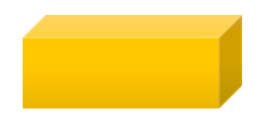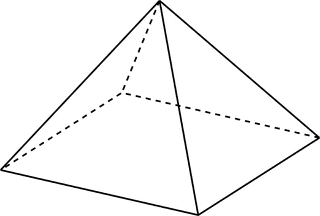# Geometry

## Objective

Identify and describe two-dimensional figures that result from slicing three-dimensional figures.

## Common Core Standards

### Core Standards

?

• 7.G.A.3 — Describe the two-dimensional figures that result from slicing three-dimensional figures, as in plane sections of right rectangular prisms and right rectangular pyramids.

?

• 5.G.B.3

• 5.MD.C.3

## Criteria for Success

?

1. Understand that a three-dimensional figure can be sliced in various ways that result in a two-dimensional cross-section
2. Understand that a slice through a right pyramid or prism, parallel to the base, will have the same shape as the base.
3. Identify the two-dimensional figures that result from various slices through right pyramids and prisms.

## Tips for Teachers

?

Visualizing the two-dimensional figures that result from slicing a three-dimensional figure can be challenging for some students. The following are two visual supports:

#### Fishtank Plus

• Problem Set
• Student Handout Editor
• Vocabulary Package

## Anchor Problems

?

### Problem 1

Justine has a block of cheese in the shape of a square prism, as shown below.She cuts the block in half.
What are two different two-dimensional faces that could result from Justine slicing the cheese in half?

### Problem 2

A right rectangular pyramid is shown below.Describe how the following two-dimensional figures can be created by slicing the pyramid.

• rectangle
• triangle
• trapezoid

## Problem Set

?The following resources include problems and activities aligned to the objective of the lesson that can be used to create your own problem set.

?

Misha has a cube and a right-square pyramid that are made of clay. She placed both clay figures on a flat surface.

Misha will make slices through each figure that are parallel and perpendicular to the flat surface. Which statements are true about the two-dimensional plane sections that could result from one of these slices?

Select all that apply.

1. A plane section that is triangular could result from one of these slices through the cube.
2. A plane section that is square could result from one of these slices through the cube.
3. A plane section that is rectangular but not square could result from one of these slices through the cube.
4. A plane section that is triangular could result from one of these slices through the pyramid.
5. A plane section that is square could result from one of these slices through the pyramid.
6. A plane section that is rectangular but not square could result from one of these slices through the pyramid.

#### References

PARCC Mathematics Practice Tests Grade 7 Paper-Based Practice TestQuestion #20